Introduction to Arithmetic Progression - Arithmetic Progressions, CBSE, Class 10, Mathematics

# Introduction to Arithmetic Progression - Arithmetic Progressions, CBSE, Class 10, Mathematics - Notes - Class 10

 1 Crore+ students have signed up on EduRev. Have you?

INTRODUCTION
Consider the following arrangement of numbers :
(i) 1, 3, 5, 7, .......

(ii) 3, 6, 12, 24, .......

(iii) 1, 4, 9, 16, .......

In each of the above arrangements, we observe some patterns. In (i) we find that the succeeding terms are obtained by adding a fixed number [i.e. 2], in (ii) by multiplying with a fixed number [i.e. 2], in (iii) we find that they are squares of natural numbers.
In this chapter, we shall discuss one of these patterns in which succeeding terms are obtained by adding a fixed number to the preceding terms. We shall also see how to find their nth terms and the sum of n consecutive terms, and use this knowledge in solving some daily life problems.

HISTORICAL FACTS

Gauss was a very talented and gifted mathematician of 19th century who developed the formula :

1 + 2 + 3 + 4 +.... + (n – 1) + n =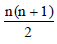for the sum of first n natural numbers at the age of 10.

He did this in the following way :
S = 1 + 2 + 3 + ... + (n – 2) + (n – 1) + n

S = n + (n – 1) + (n – 2) +.....+ 3 + 2 + 1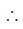2S = (n + 1) + (n + 1) + (n + 1) +....+ (n + 1) + (n + 1) + (n + 1)

= (n + 1) (1 + 1 + 1 +... upto n times)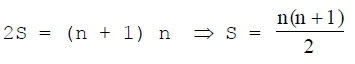Even when he was a little child of three he could read and make mathematical calculation himself. Gauss provedthe fundamental theorem of Algebra when he was 20 years old. His contribution to mathematics has been immense because his formulae were used in applied field of Astronomy, Differential Geometry and Electricity widely all over the world by scientists.

SEQUENCE
In our daily life, we come across the arrangement of numbers or objects in an order such as arrangement of students in a row as per their roll numbers, arrangement of books in the library, etc.
An arrangement of numbers depends on the given rule :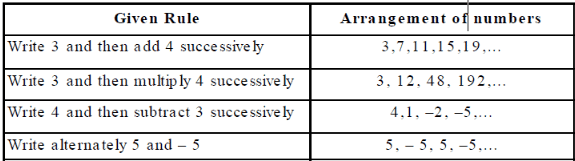Thus, a sequence is an ordered arrangement of numbers according to a given rule.
Terms of a Sequence : The individual numbers that form a sequence are the terms of a sequence.
For example : 2, 4, 6, 8, 10,.... forming a sequence are called the first, second, third, fourth and fifth,.... terms of the sequence.
The terms of a sequence in successive order is denoted by 'T'n or 'a'n. The nth term T'n is called the general term of the sequence.

SERIES
The sum of terms of a sequence is called the series of the corresponding sequence.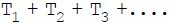is an infinite series, where as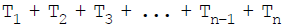is a finite series of n terms.

Usually the series of finite number of n terms is denoted by Sn.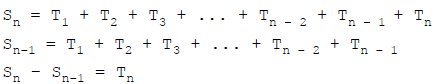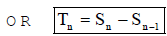Ex.1 Write the first five terms of the sequence, whose nth term is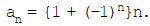Sol.Substituting n = 1, 2, 3, 4 and 5, we get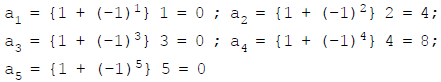Thus, the required terms are : 0, 4, 0, 8 and 0.

Ex.2  Find the 20th term of the sequence whose nth term is,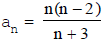Sol.Putting n = 20, we obtain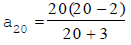Thus,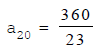Ex.3 The fibonacci sequence is defined by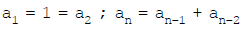for n > 2. Find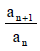, for n = 1, 2, 3, 4, 5.
Sol. We have a1 = a2 = 1 andSubstituting n = 3, 4, 5 and 6, we get.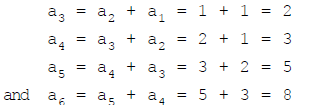Now, we have to findfor n = 1, 2, 3, 4 and 5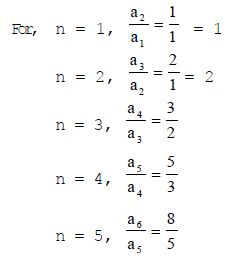Hence, the required values are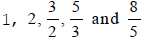COMPETITION WINDOW

SERIES OF NATURAL NUMBERS

1. The sum of first n natural numbers i.e. 1 + 2 + 3 +....+ n is usually written as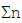.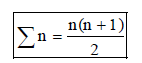2. The sum of squares of first n natural numbers i.e.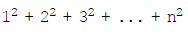is usually written as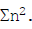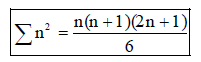3. The sum of cubes of first n natural numbers i.e.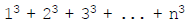is usually written as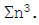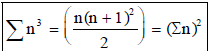PROGRESSION
It is not always possible to write each and every sequence of some rule.
For example : Sequence of prime numbers 2, 3, 5, 7, 11,... cannot be expressed explicitly by stating a rule and we do not have any expression for writting the general term of this sequence.
The sequence that follows a certain pattern is called a progression. Thus, the sequence 2, 3, 5, 7, 11,.... is not a progression. In a progression, we can always write the nth term.
Consider the following collection of numbers :
(i) 1, 3, 5, 7...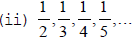From the above collection of numbers, we observe that

1. Each term is greater than the previous by 2.

2. In each term the numerator is 1 and the denominator is obtained by adding 1 to the preceding denominator.
Thus, we observe that the collection of numbers given in (i) and (ii) follow a certain pattern and as such are all progressions.

ARITHMETIC PROGRESSIONS

An arithmetic progression is that list of numbers in which the first term is given and each term, other than the first term is obtained by adding a fixed number 'd' to the preceding term.

The fixed term 'd' is known as the common difference of the arithmetic progression. It's value can be positive negative or zero. The first term is denoted by 'a' or 'a1' and the last term by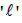.

Ex. Consider a sequence 6, 10, 14, 18, 22, .....

Here,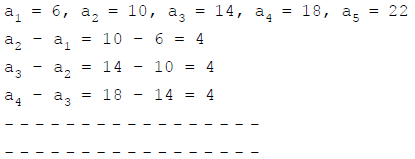Therefore, the sequence is an arithmetic progression in which the first term a = 6 and the common difference d = 4.

Symbolical form : Let us denote the first term of an AP by a1, second term by a2, .... nth term by an and the common difference by d. Then the AP becomes a1, a2, a3,....,an.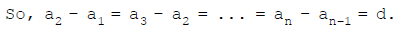General form : In general form, an arithmetic progression with first term 'a' and common difference 'd' can be represented as follows :
a, a + d, a + 2d, a + 3d, a + 4d,....

Finite AP : An AP in which there are only a finite number of terms is called a finite AP. It may be noted that each such AP has a last term.
Ex. (a) The heights (in cm) of some students of a school standing in a queue in the morning assembly are 147, 148, 149,..., 157.
(b) The minimum temperatures (in degree Celsius) recorded for a week in the month of January in a city arranged in ascending order are –3.1, – 3.0, –2.9, – 2.8, – 2.7, – 2.6, – 2.5
Infinite AP : An AP in which the number of terms is not finite is called infinite AP. It is note worthy that such APs do not have a last term.

Ex. (a) 1, 2, 3, 4,......
(b) 100, 70, 40, 10,.....

Least Information Required : To know about an AP, the minimum information we need to know is to know both – the first term a and the common difference d.
For instance if the first term a is 6 and the common difference d is 3, then AP is 6, 9, 12, 15,...
Similarly, when
a = – 7, d = – 2, the AP is –7, –9, –11, –13,...
a = 1.0, d = 0.1, the AP is 1.0, 1.1, 1.2, 1.3,...
So if we know what a and d are we can list the AP.

Ex.4 Write first four terms of the AP, when the first term a and the common difference d are given as follows :
(i) a = 4, d = 5

(ii) a = – 1.25, d = – 0.25

Sol. (i) a = 4, d = 5
First term, a = 4
Second term = 4 + d = 4 + 5 = 9
Third term = 9 + d = 9 + 5 = 14
Fourth term = 14 + d = 14 + 5 = 19
Hence, first four terms of the given AP are 4, 9, 14, 19.
(ii) a = – 1.25, d = – 0.25
First term = a = – 1.25
Second term = – 1.25 + d = – 1.25 + (– 0.25) = – 1.50
Third term = – 1.50 + d = – 1.50 + (– 0.25) = – 1.75
Fourth term = – 1.75 + d = – 1.75 + (– 0.25) = – 2.00
Hence, first four terms of the given AP are –1.25, – 1.50, – 1.75, – 2.00

Ex.6 For the AP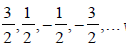write the first term a and the common difference d. Also write the next two terms after the given last term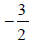Sol.  We have,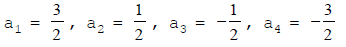and so on.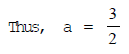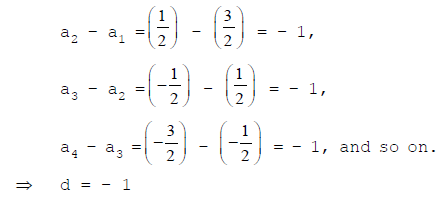Now, we find the successor of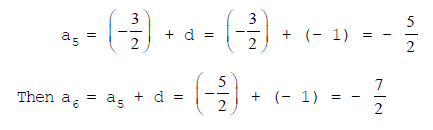Hence, the next two terms after the given termare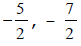COMPETITION WINDOW

GEOMETRIC PROGRESSION

1. A sequence of non-zero numbers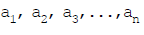is said to be a geometric sequence or G.P.

if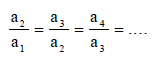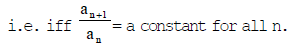This constant is called the common ratio of the G.P. and is usually denoted by 'r'. e.g., 3, 9, 27, 81,....
A general G.P. is a, ar, ar2,....
When the terms of a geometric sequence are added, we get a geometric series.

HARMONIC PROGRESSION

A sequence of non-zero numbersis said to be a harmonic sequence or H.P.

if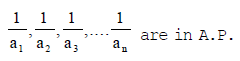e.g., (i) 12, 6, 4, 3.....

(ii) 10, 30, –30, –10, –6,....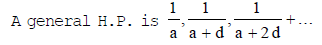where a is the first term and d is the common difference of the A.P.

GENERAL TERM OF AN ARITHMETIC PROGRESSION

The formula for writing general term or the nth term of an arithmetic progression is
an = a + (n – 1)d
Where, a is the first term of arithmetic progression, and d is the common difference of arithmetic progression.

rth TERM OF A FINITE ARITHMETIC PROGRESSION FROM THE END

Let there be an arithmetic progression with first term a and common difference d. If there are n terms in the arithmetic progression, then rth term from the end = a + (n – r)d
Also, if l is the last term of the arithmetic progression then rth term from the end is the rth term of an arithmetic progression whose first term is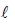and common difference is – d.
rth term from the end =+ (r – 1) (– d)

Ex.7 The 6th term of an arithmetic progression is – 10 and the 10th term is – 26. Determine the 15th term of the AP.
Sol. Let the first term and the common difference of the AP be a and d respectively.
6th term = – 10 (Given)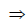a + (6 – 1)d = – 10                     [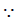an = a + (n – 1) d]a + 5d = – 10 ...(i)
10th term = – 26 (Given)a + (10 – 1)d = – 26a + 9d = – 26 ...(ii)
Solving (i) and (ii) we get
a = 10, d = – 4
Therefore, 15th term of the AP
= a + (15 – 1)d                           [an = a + (n – 1) d]
= a + 14d
= 10 + 14 (–4)
= 10 – 56 = – 46
Hence, the 15th term of AP is – 46.

Ex.8  Find the 6th term from the end of the AP 17, 14, 11,...., – 40.
Sol. The given AP 17, 14, 11,..., – 40
Here, a = 17, d = 14 – 17 = – 3,= – 40
Let there be n terms in the given AP.
Then, nth term = – 40a + (n – 1)d = – 40 [an = a + (n – 1) d]17 + (n – 1) (–3) = – 40(n – 1) (–3) = – 40 – 17(n – 1) (–3) = – 57n – 1 =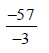n – 1 = 19n = 19 + 1n = 20
Hence, there are 20 terms in the given AP. Now, 6th term from the end
= a + (20 – 6)d                                   [rth term from the end = a + (n – r)d]
= a + 14d
= 17 + 14(–3)
= 17 – 42 = – 25
Hence, the 6th term from the end of the given AP is – 25.

Sol. The given sequence is 3, 7, 11, 15,...
a2 – a1 = 7 – 3 = 4
a3 – a2 = 11 – 7 = 4

a4 – a3 = 15 – 11 = 4
As aK+1 – ak is the same for k = 1, 2, 3, etc., the given sequence form an AP.
Here, a = 3, d = 4
Let 200 be the nth term of the given sequence. Then,
an = 200a + (n – 1)d = 2003 + (n – 1) 4 = 200(n – 1) =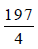n =+ 1n =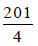But n should be a positive integer. So, 200 is not any term of the given sequence.

The document Introduction to Arithmetic Progression - Arithmetic Progressions, CBSE, Class 10, Mathematics - Notes - Class 10 is a part of Class 10 category.
All you need of Class 10 at this link: Class 10

Track your progress, build streaks, highlight & save important lessons and more!

,

,

,

,

,

,

,

,

,

,

,

,

,

,

,

,

,

,

,

,

,

,

,

,

,

,

,

,

,

,

;